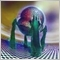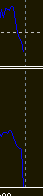RSI based indicator with value going to 0 for every new candle1611

Hi, thanks for being present in here.
I ordered an Indicator based on RSI that load everything like it should, but when a new candle opens the new RSI value go to 0. Sometimes it back to normal, some times don't. What should i look on code to fix that?
I asked developer to fix, but he sent me a file with the same problem.
I don't know how to code, but i understand the idea. I found that :

//B = Maximum_History_Bar;
//if(B>iBars(Symbol(),PERIOD_CURRENT))
B = iBars(Symbol(),PERIOD_CURRENT);
if(B==0)
B = Maximum_History_Bar;
rsibuffer[B-1] = rsiarray[B-1];
//rsibuffer[B] = rsiarray[B];
int    ContinuesCounter = 0;
for(int i=2; i<B; i++)
{
//rsibuffer[B-i] = rsiarray[B-i];
if(closearray[B-i]<closearray[B-(i+1)] && closearray[B-i]<closearray[B-(i-1)])
//if(lowarray[B-i]<lowarray[B-(i+1)] && lowarray[B-i]<lowarray[B-(i-1)])
{
double FirstRSI  = rsiarray[B-i];
ContinuesCounter = 0;
DiverganceStrong = 0;

There's anything in here that could be changed to fix my problem? Or what in the code should i search?

Also, the indicator draw lines over the RSI Window, and these lines are getting misaligned when a candle appear, seems like RSI move more than the lines do (or maybe they don't move).

What in the code should make it move correctly?

I would love to get help from the community, but I can't just upload the code in here.
Thanks, i'm here everyday, the whole day as i'm working hard on a EA Based on that Indicator. And for now, it's almost impossible to delete and add again this indicator for every new Candle.
although it keep drawing lines as it was meant to do, I just can figure out where on the RSI values theses lines are.1611

I tried reading documentations about "making an indicator based on another indicator" And i can't find in my code where is missing the lines that should update the RSI Value.

Just to illustrate :Standard RSI
____________

My code